• C语言代码，自学C语言时，都是自己打的，欢迎大家下载学习
• C语言简单代码程序 注释： C语言中 // 用于行注释。 例： // printf("此行为注释,编译运行时忽略此句"); /**/用于段落注释 例： /* printf("此处为段落注释"); */ 标准输入输出程序(顺序结构)： #include<...
C语言简单代码程序
注释：
C语言中  //  用于行注释。
例：
// printf("此行为注释,编译运行时忽略此句");

/**/用于段落注释
例：
/*
printf("此处为段落注释");
*/

标准输入输出程序(顺序结构)：

#include<stdio.h>          //编译预处理， .h为头文件，本句相当于将 stdio.h文件里的所有内容

int main(void)            //主函数   main()函数
{
int num;              //设置一个变量，用来储存整数数据
scanf("%d" ,&num);    //调用标准输入文件scanf()输入整数给num变量
printf("%d", num);    //调用标准输出文件printf()将num中的数据输出
return 0;
}

分支结构：
//  此程序用于输出两个数中的最大值
#include<stdio.h>

int main(void)            //主函数   main()函数
{
int num1 = 4, num2 = 5;              //设置两个变量并进行初始化，为其赋值
if(num1 > num2)
{
printf("%d", num1);
}
else
{
printf("%d", num2);
}
return 0;
}

循环结构：
//此处用于输出1~100的数
#include<stdio.h>

int main(void)            //主函数   main()函数
{
int i;
for(i = 1; i <= 100; i++)    //for循环，变量i 从 1 到 100
{
printf("%d\n",i);
}
return 0;
}

刚开始学c语言的同学们慢慢来，多多动手敲代码是很有效的学习方式！


展开全文• ## 爱心c语言简单代码

千次阅读 多人点赞 2020-05-16 16:38:06
前言：对于c语言，在我看来十分重要，是所有语言编程语言的鼻祖。 今天先跟大家分享一个编程c语言的软件，visual ...今天给大家分享一个爱心的代码： #include<stdio.h> void a(int aa) { for (int i = 0; i &l
前言：对于c语言，在我看来十分重要，是所有语言编程语言的鼻祖。
今天先跟大家分享一个编程c语言的软件，visual studio 2019在这里选择你想要的版本。
安装密匙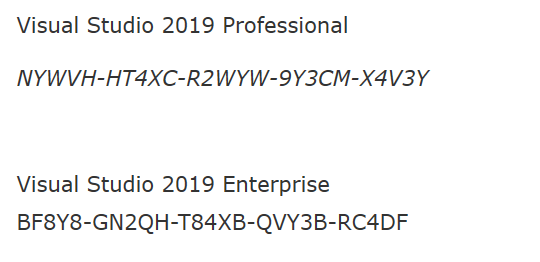这是一些，希望可以帮助到大家。
安装以后我们打开vs2019，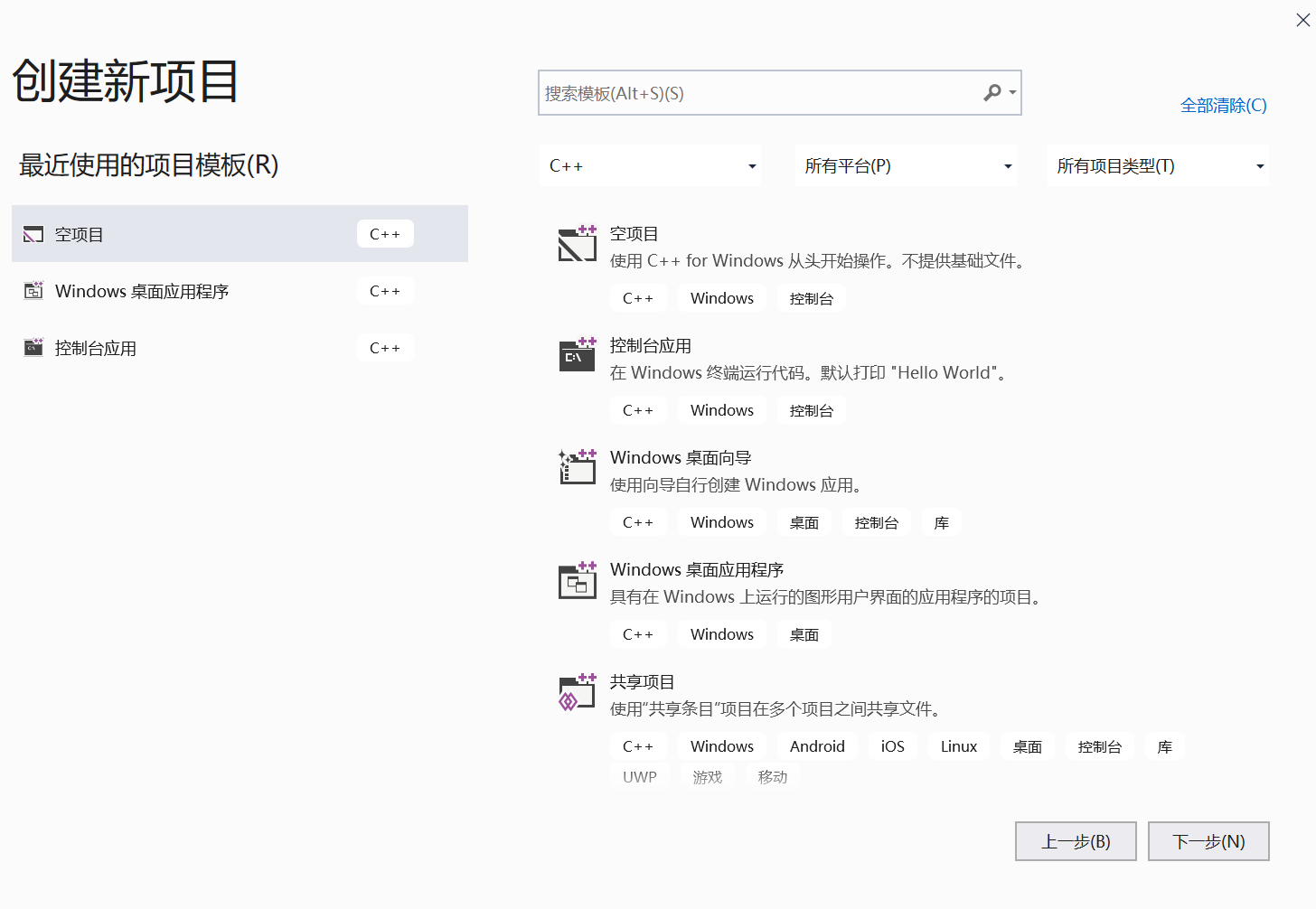创建一个空项目。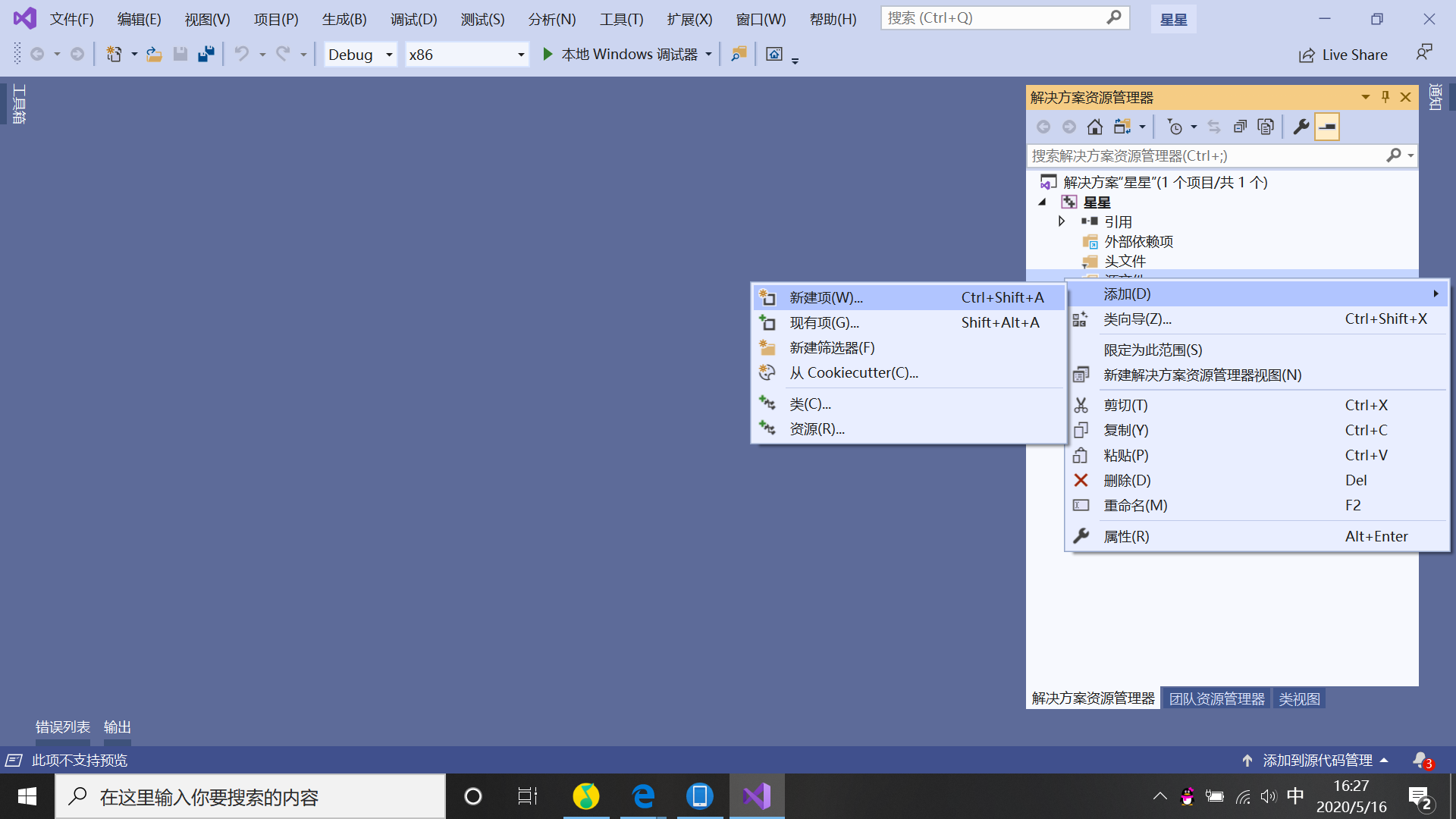在源文件里，添加一个新建项，选择带.h的头文件。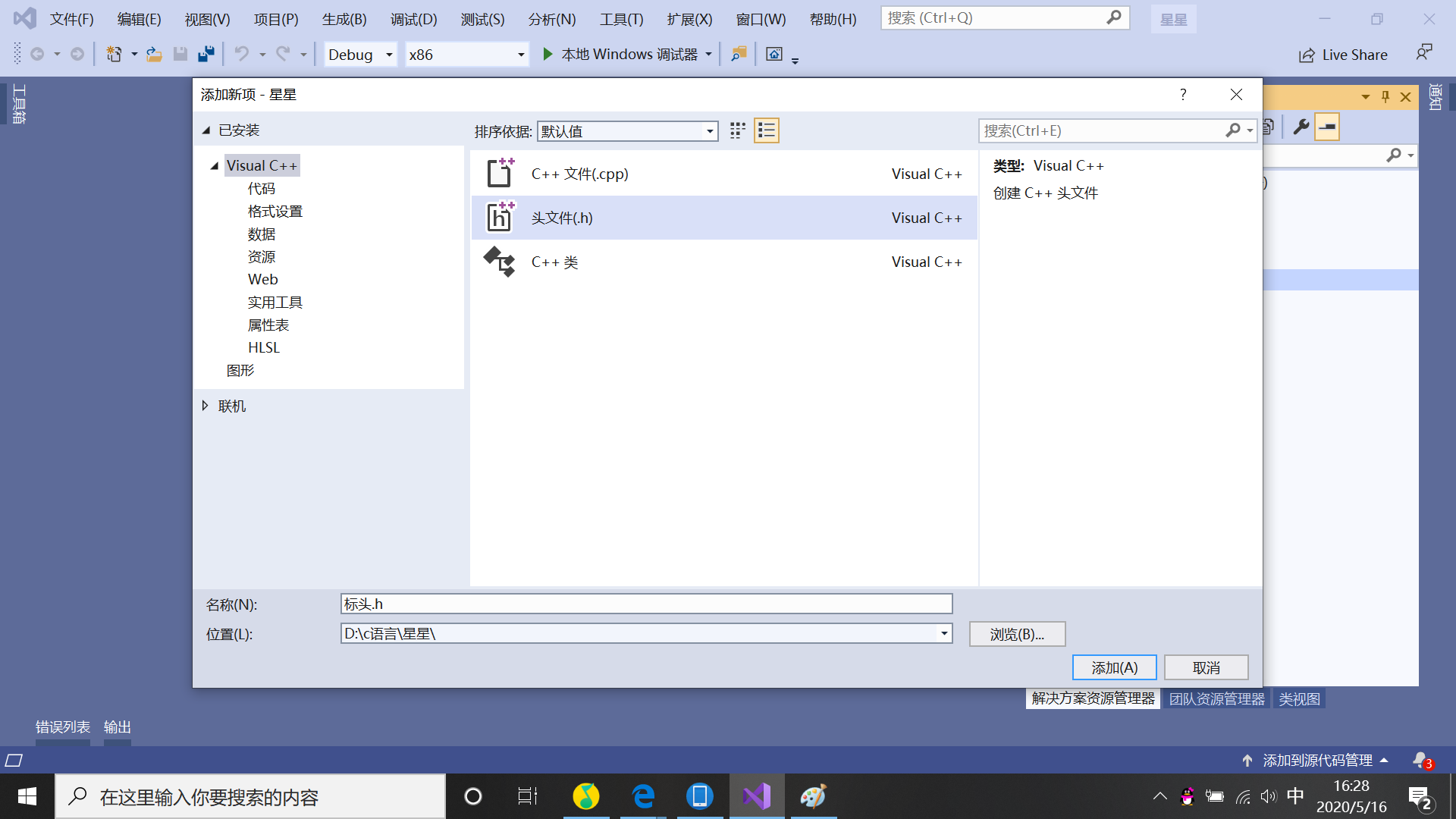这样就可以开始愉快的c语言编程了。
今天给大家分享一个爱心的代码：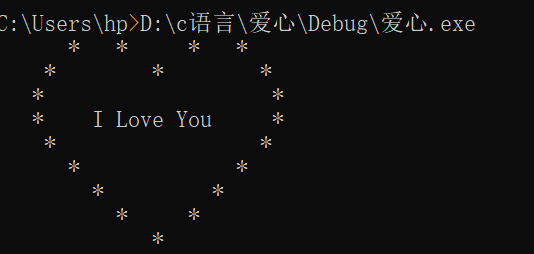#include<stdio.h>
void a(int aa)
{
for (int i = 0; i < aa; i++)printf(" ");
}
void b()
{
printf("*");
}
char c(int cc)
{
printf("%c", cc); return 0;
}
int main()
{
a(6); b(); a(3); b(); a(5); b(); a(3); b();
printf("\n");
a(4); b(); a(8); b(); a(8); b();
printf("\n");
a(3); b(); a(19); b();
printf("\n");
a(3); b(); a(4); c(73); a(1); c(76); c(111); c(118);
c(101); a(1); c(89); c(111); c(117); a(5); b();
printf("\n");
a(4); b(); a(17); b();
printf("\n");
a(6); b(); a(13); b();
printf("\n");
a(8); b(); a(9); b();
printf("\n");
a(10); b(); a(5); b();
printf("\n");
a(13); b();
printf("\n");
getchar();
return 0;

***

> 小编也不容易，喜欢的点个赞！！！

***



展开全文• #include <stdio.h> #include using namespace std; int i,k; //char *s1,*s2; void linear(double *data1,int *data2,double &w,double &b) { int j,t; ...fp1= fopen(“E:\data...
#include <stdio.h>
#include
using namespace std;
int i,k;
//char *s1,*s2;
void linear(double *data1,int *data2,double &w,double &b)
{
int j,t;
k=0,i=0;
FILE *fp,*fp1;
fp1= fopen(“E:\data\data1.txt”, “r”);
fp = fopen(“E:\data\data2.txt”, “r”);
if(fp == NULL|fp1==NULL)
cout<<“false”;
while(fscanf(fp, “%d”, &data2[i]) != EOF)
i++;
while(fscanf(fp1, “%lf”, &data1[k]) != EOF)
k++;
fclose(fp);fclose(fp1);
cout<<“获取的数据集为：”<<endl;
cout<<“xi”<<"                            “<<“yi”<<endl;
for(j=0;j<i;j++){
cout<<data1[j]<<”                           "<<data2[j]<<endl;
}
 double sum=0,average;

for(t=0;t<k;t++){
sum+=data1[t];
}
average=sum/j;
double Molecular=0;
for(j=0;j<i;j++){
Molecular+=data2[j]*(data1[j]-average);
}
double sum1=0;
for(j=0;j<i;j++){
sum1+=data1[j]*data1[j];
}
double j1=(double)1/j;
double denominator=sum1-j1*sum*sum;
w=Molecular/denominator;
double sumb=0;
for(j=0;j<i;j++){
sumb+=(data2[j]-w*data1[j]);
}
//cout<<endl<<sumb<<" "<<j1;
b=j1*sumb;

}
int main(){
double data1;int data2;
double w, b;
linear(data1,data2,w,b);
cout<<“通过最小二乘法求得参数：”<<endl<<“w=”<<w<<endl<<“b=”<<b;
cout<<endl<<“通过数据集学的的线性模型为：”<<endl<<“f(x)=”<<w<<“x+”<<b;
double x;
while(cin>>x){
cout<<"x值为： "<<x<<endl;
cout<<"预测值为： " <<w*x+b;
}
}


展开全文• 花了一个小时写了一个最基本的飞机大战小游戏，代码还可以完善很多，许多玩法还没有加，最近学校开课了一直在上网课，等有时间了会再把这个代码去完善一下，各位大佬也可以给我提出一些建议。 #include<stdio.h&...
花了一个小时写了一个最基本的飞机大战小游戏，代码还可以完善很多，许多玩法还没有加，最近学校开课了一直在上网课，等有时间了会再把这个代码去完善一下，各位大佬也可以给我提出一些建议。
#include<stdio.h>
#include<windows.h>
#include<conio.h>
#include<time.h>
#pragma warning(disable:4996)
int main()
{
int x = 20;
int y = 20;
int i = 0;
int j = 0;
int nx = 5;
int killed = 0;
int isFird = 0;
char input;
while (1)
{
system("cls");
if (killed == 0)
{
for (i = 0; i < nx; i++)
{
printf("  ");
}
printf("+");
}
if (isFird == 0)
{
for (i = 0; i < y; i++)
{
printf("\n");
}
}
else
{
for (i = 0; i < y; i++)
{
for (j = 0; j < x; j++)
{
printf("  ");
}
printf("   |\n");
}
if ((x + 3) == nx)
{
killed = 1;
}
isFird = 0;
}
for (i = 0; i < x; i++)
{
printf("  ");
}
printf("   *\n");
for (i = 0; i < x; i++)
{
printf("  ");
}
printf("*******\n");
for (i = 0; i < x; i++)
{
printf("  ");
}
printf(" *****\n");
for (i = 0; i < x; i++)
{
printf("  ");
}
printf("  * *\n");
input = getch();
if (input == 'w')
{
y--;
}
else if (input == 's')
{
y++;
}
else if (input == 'a')
{
x--;
}
else if (input == 'd')
{
x++;
}
else if (input == ' ')
{
isFird = 1;
}
else
{
printf("输入错误，请重新输入!!!\n");
continue;
}
}
system("pause");
return 0;
}



展开全文•c 语言
• 但是代码之路永无止境，今天我们来看看1-100循环和代码，比较简单，但是想明白以后循环你也能入门了，首先放源码，需要的看下面，有注释还有其他写法 //这是标准写法 下面有注释版本 #include<stdio.h> int ...算法
• 利用c语言实现简单心形的代码分享发布时间：2020-03-24 14:40:02来源：亿速云阅读：1451作者：小新今天小编要说的...c语言简单心形代码用c语言，制作一个心形图案。送给c语言初学者。代码如下：#include int main() ...
•编译器
• 一个简单C语言病毒代码，供其他初学C语言的同学参考
• 贺卡代码 C语言的一个贺卡代码 用Visual Studio打开
• 双人贪吃蛇，别踩白方块，玫瑰花，矿井逃生等多种C语言游戏代码等你来实践！
• C语言编写的简易五子棋程序，内附简易操作规则，适合初学者学习和使用。学习C语言程序代码能够在VC 6.0或者更高版本的运行环境下运行。
• 单片机学习，STC90C51开发版简易电子琴制作C语言代码
• 这是扫雷的源代码C语言编的 一些常用小软件的源...这是扫雷的源代码C语言编的 一些常用小软件的源代码这是扫雷的源代码C语言编的 一些常用小软件的源代码这是扫雷的源代码C语言编的 一些常用小软件的源代码
• 此程序能实现学生记录的录入，学生成绩管理，学生记录的查找、追加、删除、修改、保存，教学计划的制定等。 此程序将整个教务系统划分成两部分，即学生管理和课程管理。...（详细参考《零点起飞学C语言》）
• 初学C语言,自己编写了一个贪吃蛇的小代码,会的不多,代码简单,希望初学的C语言的通知能有所借鉴,也希望大佬多批评指正.谢谢!
• 关于简易的整数计算机的设计制作算是c语言中较为基础的一道题，之前刚上课时老师也介绍过，但是我是初学，时间久了容易忘记，所以在此记录一下。 #include int main() { int a,op,b; scanf("%d%c%d",&a,&op,&b);...
• ## C语言病毒代码

千次阅读 2013-12-05 10:39:54
在下面的部分加上传染部分就可以了，比如... C语言病毒代码  #include #include #include #include #include /* copy outfile to infile */ void copyfile(char *infile, char *
• 五子棋C语言程序代码 #include <graphics.h> #include <stdio.h> #include <conio.h> void drawPanel(); int isWin(int,int); int color = 1; //1-红色 2-白色 int chessman; //主函数...
• c语言简单病毒自我复制代码c语言简单病毒自我复制代码c语言简单病毒自我复制代码c语言简单病毒自我复制代码
• 一个简易的、用C语言写的五子棋游戏源代码，仅供参考！
• ## C语言 爱心代码

千次阅读 2020-06-09 22:05:35
需要的自取，看明白了就可以自己修改爱心的大小 #include<stdio.h> int main() { int n,k,m,p,q; for(n=1; n<=2; n++) { for(k=1; k<=15-n; k++) printf(" "); for(m=1;...=5-2*
• ## c语言单链表代码

万次阅读 2019-04-20 22:54:09
// 操作系统 win 8.1 // 编译环境 Visual Stuido 2017 #include<stdio.h> #include<malloc.h> #include<stdlib.h>...typedef int ElementType; // 定义数据类型,可根据需要进行其他类型定义 ......

# c语言简单代码c语言 订阅